# Folded Counterpoise Systems

## Analysis of standard, loaded, and folded Counterpoise Systems

is not complete at present, and will eventually be split into linear loading and
counterpoise pages. I tried to model the FCP under a 45-50 foot high inverted L.
My thinking was by varying height we could see if the FCP divorced earth from
the counterpoise as proposed. That didn’t work out well, I found any sparse or
very high angles. When I move the L antenna and radial system up above earth,
the system starts to act like a low dipole and have very strong radiation from
the flattop. This makes efficiency look dramatically better in short or sparse
radial systems with increased height above earth, but the bulk of “new” EM
energy is at very high angles. My next efforts will be repeating the models and
comparing estimated field strength at lower angles. See table at end.

## Comparison Table Using Models

While the only indisputable proof of performance come from
actual field strength comparisons between a known working reference and a system
under evaluation, models are very accurate. We must include everything
influencing the antenna, and understand simple models treat earth as a
homogeneous media. Even if a model does not perfectly represent earth and
surrounding structures, models can still provide a useful “feel” for systems
behavior.

The most frequent modeling errors are:

Not including feed lines, or analyzing the interaction of
feed lines

Not considering matching system losses

Failure to recognize models can use perfect, ground-independent,
sources

Notes:       *Unless noted, all power levels set at 1500 watts.

**”T” radials are single wires running out to a
terminating wire, just like a regular T antenna but laid flat and used as a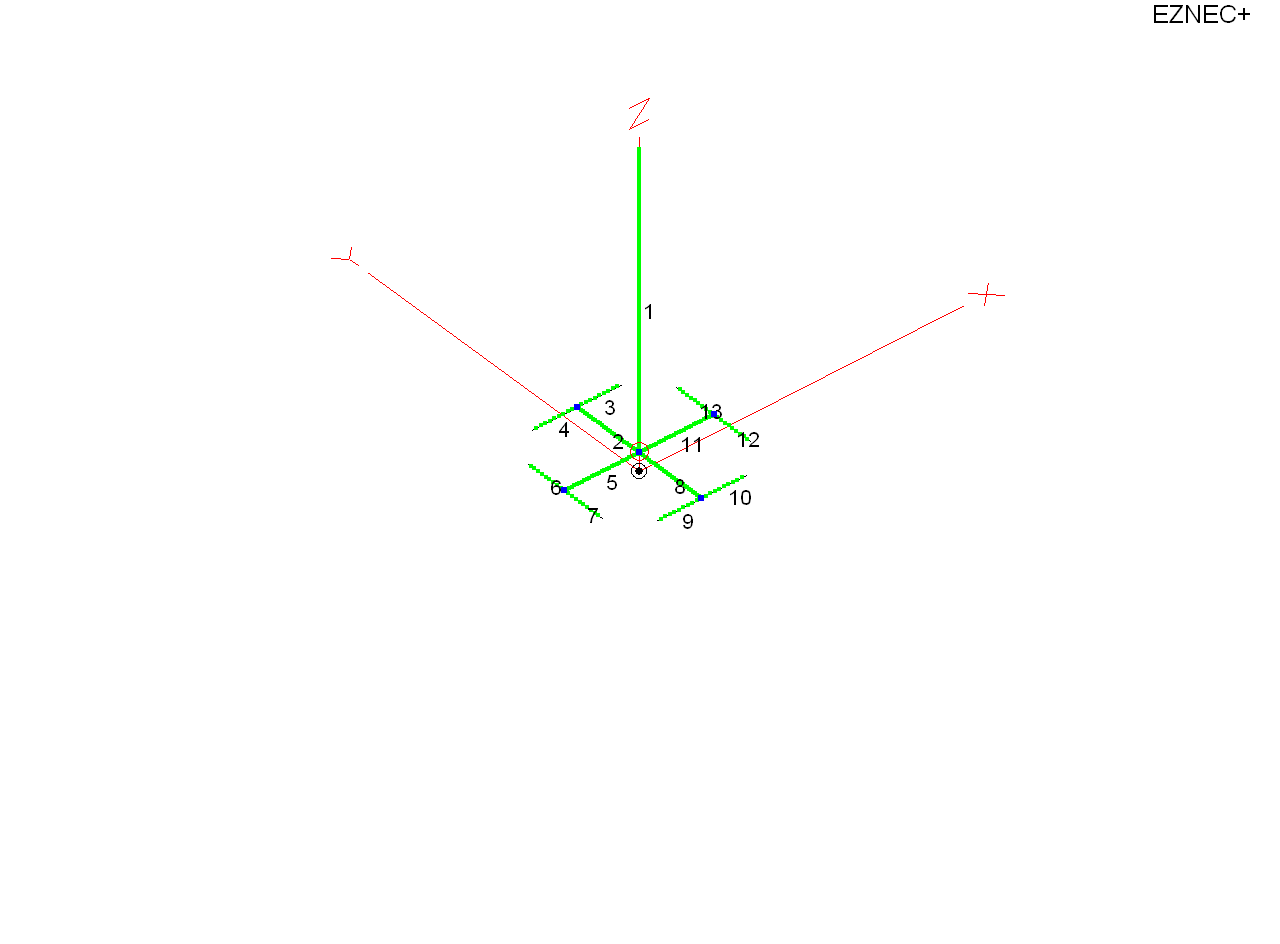## What tells us a system is behaving abnormally?

When evaluation new ideas or new systems, there are definite clues to
abnormal behavior. A few common warning signs are:

1.) Only very specific feed line coupling systems can be used. Other commonly
used or reasonably common methods fail, without logical technical explanation of
why other common methods won’t work.

2.) Claims are made models will not work, while reliable or meaningful A-B comparisons
refuting model accuracy are
missing. The common claim is “models will not work with this antenna”.

3.) Performance verifications are either comparisons of grossly dissimilar
systems (such as different polarization or antenna types), or
Gotham standard reports. How much DX we
worked, or far we worked, is not a verification of performance. I have worked
Europe, Australia, and Asia on 160 meters from my mobile, and my 160 mobile
antenna is less than 1% efficient.

4.) Comparisons are made only to less-than-ideal, or highly variable,
systems. For example, a known mediocre performance system might be used to
verify another antenna. A reference antenna must be a known, consistent,
reliable, performer. My 160 meter mobile antenna, even though less than 1%
efficient, ties or beats some large home-station antennas.

## How Do We know Counterpoise is Resonant?

One way to evaluate counterpoise resonance is by installing a known
electrical length Marconi antenna above the counterpoise. Here is a resonant
160-meter vertical over infinite perfect ground (note: current at 1 amp in
source tables):

 EZNEC+ ver. 5.0 1/4 wave vertical perfect gnd  7/31/2012 8:59:56 AM ————— SOURCE DATA ————— Frequency = 1.83 MHz Source 1 Voltage = 37.9 V at 0.24 deg. Current = 1 A at 0.0 deg. Impedance = 37.89 + J 0.1565 ohms Power = 37.89 watts SWR (50 ohm system) = 1.319 (37.9 ohm system) = 1.004

The ~1830 kHz resonant Marconi #16AWG antenna is 131.2 feet tall.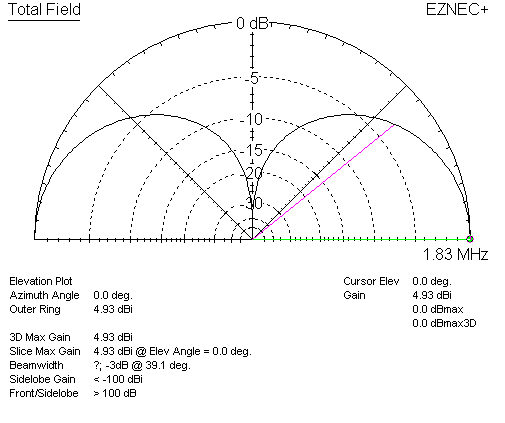We can test the electrical characteristics of a counterpoise by using the
131.2ft long Marconi antenna without any change in radiating element length. If
we place it over a ground system that is resonant, the resonate frequency of the
system should remain the same. Over the
ground system described as a “K2AV FCP” (folded counterpoise) we learn:

 EZNEC+ ver. 5.0 FCP 1/4wl vert 7/31/2012 9:26:31 AM ————— SOURCE DATA ————— Frequency = 1.83 MHz Source 1 Voltage = 198.5 V at -80.01 deg. Current = 1 A at 0.0 deg. Impedance = 34.45 – J 195.5 ohms Power = 34.45 watts SWR (50 ohm system) = 24.290 (34.45 ohm system) = 34.177

We now have ~195 ohms of capacitive reactance at the feedpoint. Clearly, the counterpoise is not resonate

With this in mind, we need to ask ourselves, “What causes
the entire system to be resonant, if the counterpoise is not resonant?”

Let’s look at the “isolation transformer”, that (according to information in
articles) cannot be replaced by anything else.

It is difficult to predict the exact effect of this transformer without
measurement, because irons cores similar to the -2 series red cores have
considerable flux leakage.
Contrary to Handbooks, they do not
“self-shield”. As a matter of fact, inductance can vary by a ratio
of 2:1 with the same number of turns spread evenly over a core, compared to the
same winding compressed in a compact core area. In many of my RF designs, when
-2 mix irons are used, stages are tuned by spreading or squeezing turns!

It has 20 turns on a T-300A2 core. This should be, with very tight
evenly-spaced turns, about +j115 ohms reactance per winding. This
considerable capacitive reactance. Use of this transformer strongly supports the
1/4λ vertical wire test model, which
indicates the counterpoise is actually resonant far above the 160-meter band.
The isolation transformer effectively adds about +j100 ohms in series with the
50 ohm feed line, and the antenna feedpoint. The exact contribution would vary
greatly from mutual coupling between windings on the much less-than-perfect
“leaky core”.

Moving the counterpoise to 1830 kHz, with 4.8 inch spacing and no dielectric,
required the following wire table: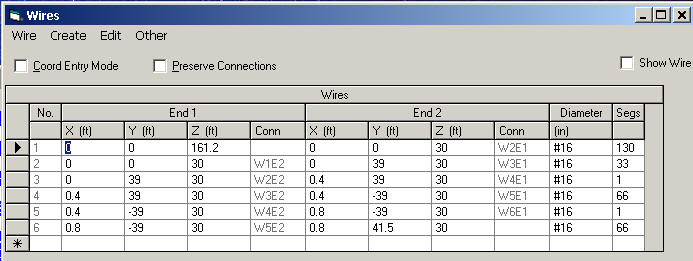After extending the counterpoise from 33 feet “radius” to 39 feet “radius”, I
adjusted the open end of wire 6 for exact resonance. This gave the following
impedance with a 131.2 foot long radiator (note: at 1 ampere base
current):

 EZNEC+ ver. 5.0 SW FCP 7/31/2012 1:18:26 PM ————— SOURCE DATA ————— Frequency = 1.83 MHz Source 1 Voltage = 39.56 V at -0.17 deg. Current = 1 A at 0.0 deg. Impedance = 39.55 – J 0.1145 ohms Power = 39.55 watts SWR (50 ohm system) = 1.264 (39.5 ohm system) = 1.003

For best performance and lowest common mode on the feeder, anyone installing
a folded loading system should tune the system in place. The general trend of
conductor for
the same resonant frequency.

Wider spacing decreases counterpoise loss, increases bandwidth, and requires
less wire length for resonance.

This is the impedance at 1-foot spacing, and the wire table for one-foot wire
spacing:

 EZNEC+ ver. 5.0 FCP 7/31/2012 1:28:28 PM ————— SOURCE DATA ————— Frequency = 1.83 MHz Source 1 Voltage = 38.1 V at -0.36 deg. Current = 1 A at 0.0 deg. Impedance = 38.09 – J 0.2403 ohms Power = 38.09 watts SWR (50 ohm system) = 1.313 (38.1 ohm system) = 1.006

Feed impedance resistive value decreased by 1.5 ohms with wider spacing,
which indicates less counterpoise loss. Impedance is a valid test in this case,
because only counterpoise spacing changed significantly, and the counterpoise
was well-above (30 feet above) lossless earth. The new wire table is: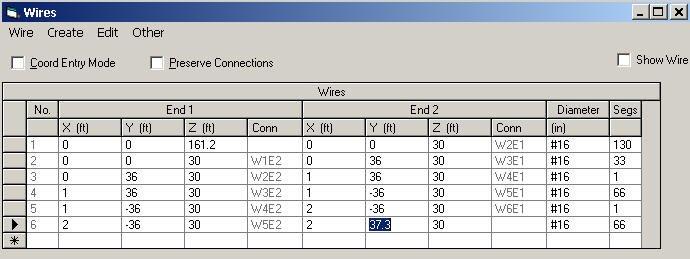Counterpoises can be measured for resonance. This is best
accomplished by using TWO identical counterpoises, in this case with them
positioned at right angles,
and measuring the counterpoises as we would a dipole. This will give a
reasonable estimate of counterpoise resonance.

We can clearly see the systems being promoted today are not even close to
resonant. The effect of non-resonance is not necessarily additional loss. The
main effect of non-resonance is change of feedpoint impedance, and an increase
in voltage between the counterpoise feed terminal and earth. Counterpoises not
occupying significant wavelength area, and sparse in radial conductors (less
than 15-30 conductors or less than .15-.2λ
long) should be isolated from earth paths. This effect, which directly relates
to counterpoise-to-earth voltage at and near the feedpoint, is aggravated when a
counterpoise is non-resonant.

 EZNEC+ ver. 5.0 one T radials 7/30/2012 11:59:05 PM  ————— SOURCE DATA ————— Frequency = 1.83 MHz Source 1 Voltage = 190.9 V at 0.16 deg. Current = 7.858 A at 0.0 deg. Impedance = 24.29 + J 0.06877 ohms Power = 1500 watts SWR (50 ohm system) = 2.058 (24.3 ohm system) = 1.003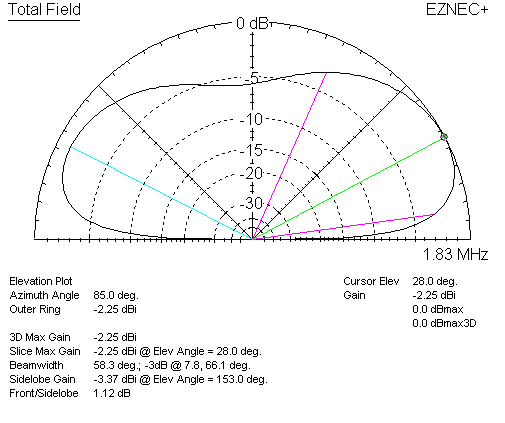EZNEC+ ver. 5.0 4x T radials 7/31/2012 12:19:22 AM ————— SOURCE DATA ————— Frequency = 1.83 MHz Source 1 Voltage = 154.9 V at -2.63 deg. Current = 9.696 A at 0.0 deg. Impedance = 15.95 – J 0.7327 ohms Power = 1500 watts SWR (50 ohm system) = 3.135 (16 ohm system) = 1.047

## Basic Inverted L with sixty 100ft long radials

 EZNEC+ ver. 5.0 Inv L 60 x 100ft radials 7/30/2012 9:49:54 PM ————— SOURCE DATA ————— Frequency = 1.83 MHz Source 1 Voltage = 172.3 V at 2.2 deg. Current = 8.713 A at 0.0 deg. Impedance = 19.76 + J 0.7595 ohms Power = 1500 watts SWR (50 ohm system) = 2.532 (19.76 ohm system) = 1.039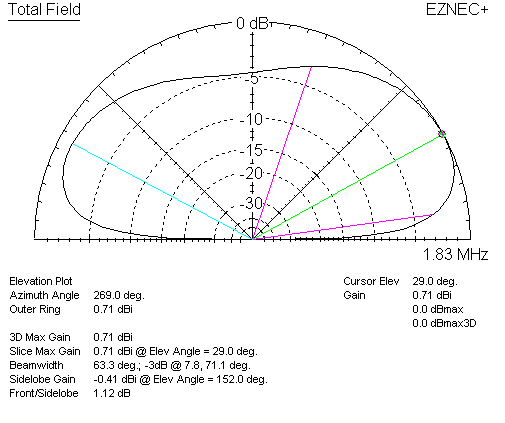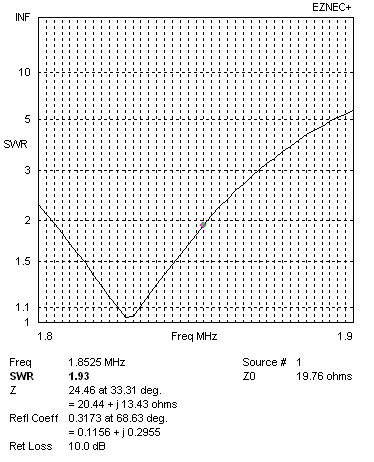2:1 SWR Bandwidth + – 25 kHz

Gain .71 dBi

19.76 ohms

58 volts radial CP to ground

## Inverted L with fifty radials fifty feet long:

 EZNEC+ ver. 5.0 Inv L 50 x 50ft radials 7/30/2012 4:43:45 PM ————— SOURCE DATA ————— Frequency = 1.83 MHz Source 1 Voltage = 198.8 V at 0.8 deg. Current = 7.546 A at 0.0 deg. Impedance = 26.34 + J 0.369 ohms Power = 1500 watts SWR (50 ohm system) = 1.898 (26.3 ohm system) = 1.014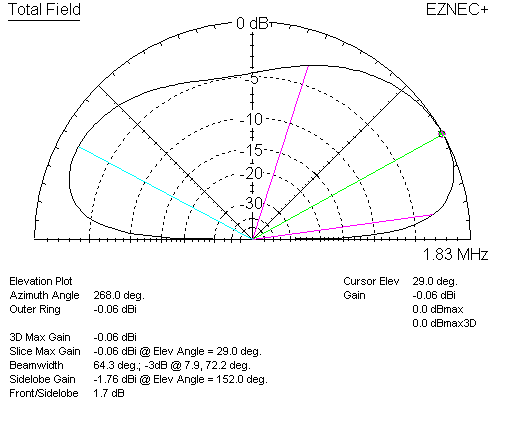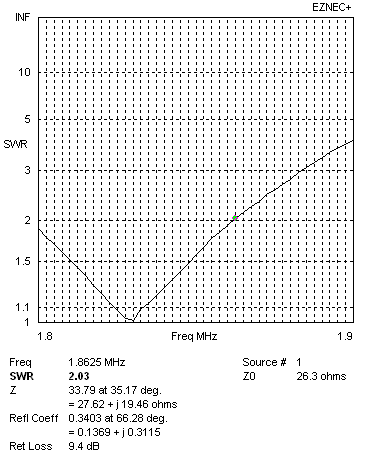2:1 SWR Bandwidth + – 30 kHz

Gain -0.06 dBi

26.3 ohms

154 volts radial CP to ground

## L antenna fifteen radials 50-feet long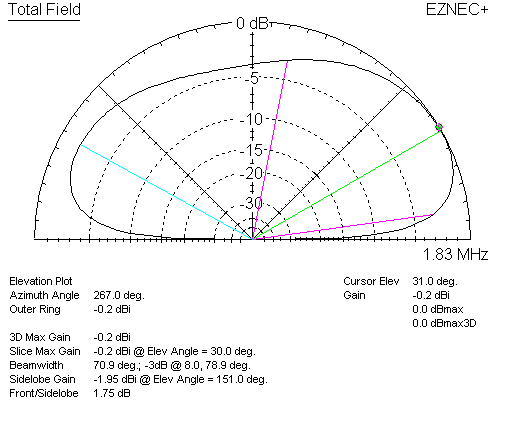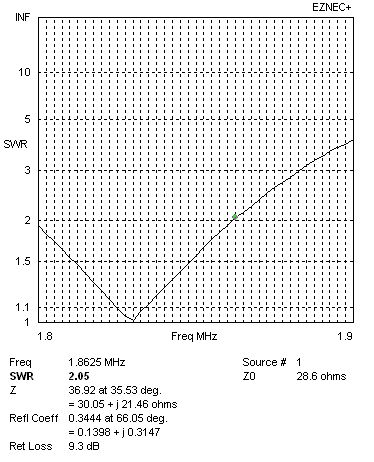2:1 SWR Bandwidth + – 30 kHz

Gain -0.2 dBi

29.5 ohms

410 volts radial CP to ground

This antenna has one radial of #16 wires spaced 1-foot. To be resonant in
folded configurations, the radial has to be 80 feet long. Inductor Q is about
220. This is a mediocre inductor, typical of #16 wire on a PVC form. To do a
direct comparison to a folded counterpoise, the resonant single-fold
counterpoise was modified to short both ends,

 EZNEC+ ver. 5.0 one resonant coil radial 7/30/2012 10:52:25 PM ————— SOURCE DATA ————— Frequency = 1.83 MHz Source 1 Voltage = 177.4 V at 0.77 deg. Current = 8.454 A at 0.0 deg. Impedance = 20.99 + J 0.2804 ohms Power = 1500 watts SWR (50 ohm system) = 2.382 (21 ohm system) = 1.013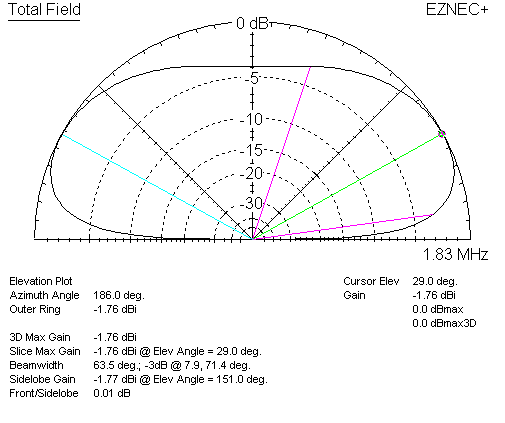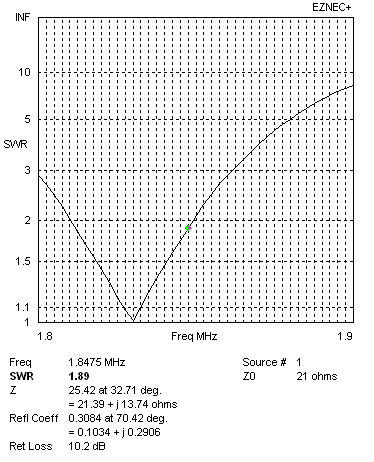2:1 SWR Bandwidth + – 18 kHz

Gain -1.76 dBi

21 ohms

143 volts radial CP to ground

### Same Inverted L Antenna with Four Inductor Loaded Radials 80-feet long

 EZNEC+ ver. 5.0 coil loaded radials x4   7/30/2012 11:07:21 PM ————— SOURCE DATA ————— Frequency = 1.83 MHz Source 1 Voltage = 166.5 V at 0.74 deg. Current = 9.011 A at 0.0 deg. Impedance = 18.47 + J 0.2388 ohms Power = 1500 watts SWR (50 ohm system) = 2.707 (18.5 ohm system) = 1.013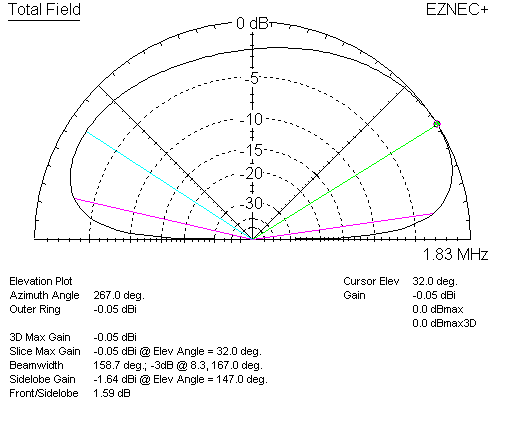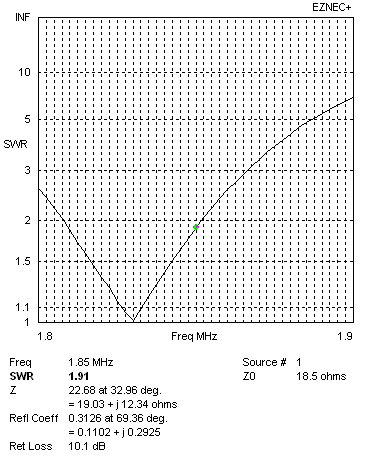2:1 SWR Bandwidth + – 22 kHz

Gain -.05 dBi

18.5 ohms

177 volts radial CP to ground

## Single Wire FCP Counterpoise

This is a single counterpoise model of an FCP system, with the base 45-feet high above
average soil. The antenna is the same basic 42-foot vertical section Inverted L
used in other models. This is slightly different than described on Internet,
because close spacing causes a guideline error warning in model. If wire spacing is
too close, a modeling rule violation warning appears. This is the closest
allowed spacing.

The effect of slightly wider fold spacing is a small downward shift in
counterpoise resonant
frequency.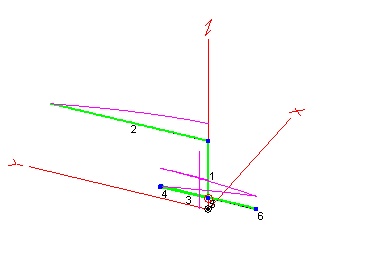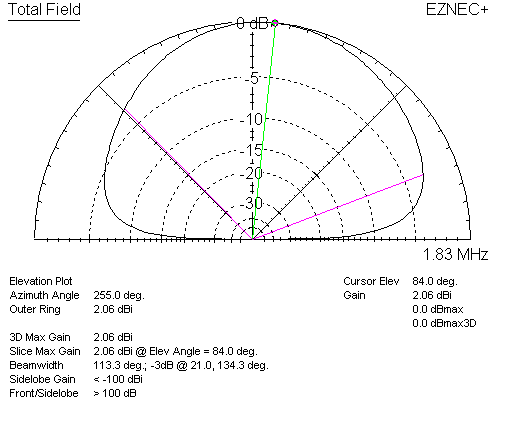Same antenna 8-feet high, L trimmed to resonance:

 EZNEC+ ver. 5.0 FCP 8ft 8/8/2012 6:41:46 PM ————— SOURCE DATA ————— Frequency = 1.83 MHz Source 1 Voltage = 209.1 V at 1.05 deg. Current = 7.176 A at 0.0 deg. Impedance = 29.13 + J 0.5349 ohms Power = 1500 watts SWR (50 ohm system) = 1.717 (29.2 ohm system) = 1.019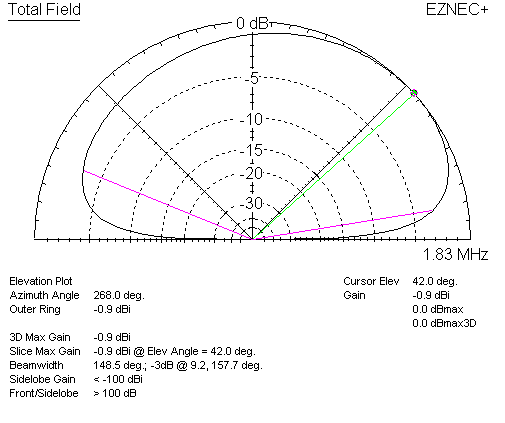2:1 SWR Bandwidth + – 18 kHz

Gain -0.9 dBi

29.13 ohms

2400 volts radial CP to ground

(efficiency 26.2%)

Same FCP antenna at 8 feet, with L horizontal at right angles to FCP: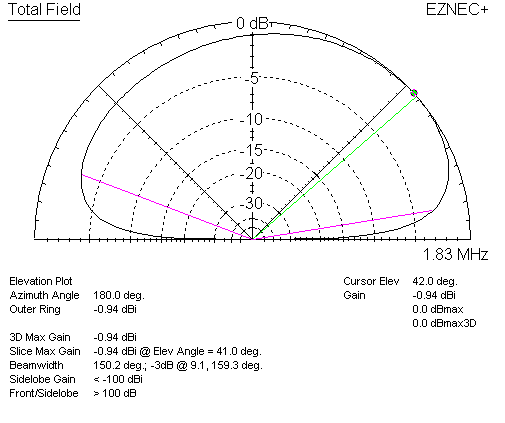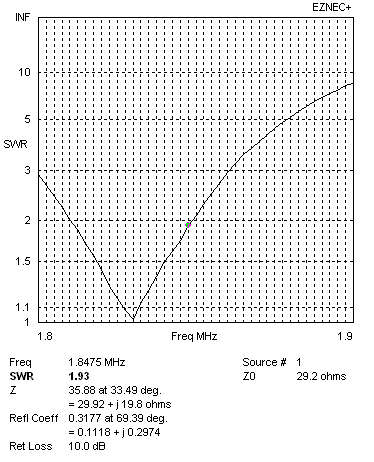## Single-fold counterpoise with Four 80-ft Counterpoise Elements

This antenna has four counterpoises made from #16 wires spaced 1-foot. To make radials
self-resonant
in single folded configurations, each radial has to be 80-feet
long. This is about 160-ft of wire in each radial. This is normal behavior of
folded wires. If radials contain only 1/4λ of total
wire length, they will not be resonant inside the band.

 EZNEC+ ver. 5.0 folded radials 7/30/2012 10:32:15 PM ————— SOURCE DATA ————— Frequency = 1.83 MHz Source 1 Voltage = 167.1 V at -1.47 deg. Current = 8.978 A at 0.0 deg. Impedance = 18.61 – J 0.4779 ohms Power = 1500 watts SWR (50 ohm system) = 2.687 (18.6 ohm system) = 1.026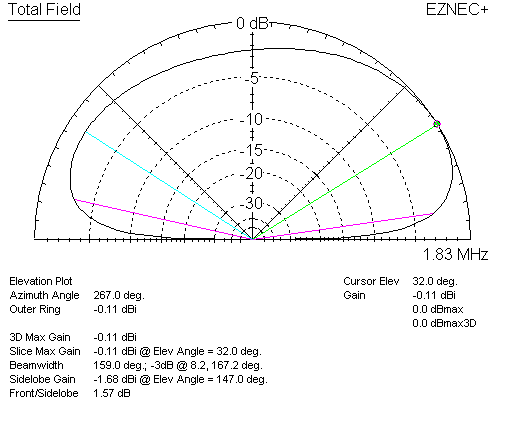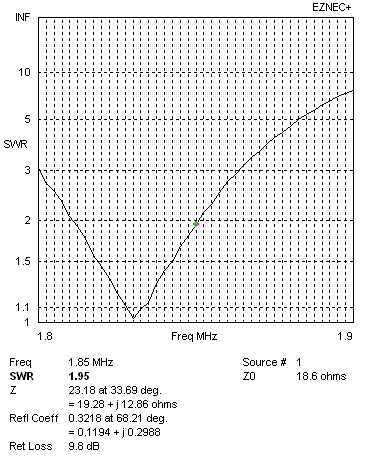2:1 SWR Bandwidth + – 20 kHz

Gain -0.11 dBi

18.6 ohms

321 volts radial CP to ground

 Antenna Inverted L 50 feet high 2:1 Bandwidth + – kHz Gain dBi Impedance ohms common point radial voltage Eff at 8 feet above earth Eff at 45 feet above earth 60 radials 100-ft long 25 .71 19.8 58 36.8% 39.1% Loaded four radials 80-ft long 22 -0.05 18.5 177 32.4% 40.4% 50 radials 50-ft long 30 -0.06 26.3 154 30.8% 45.1% folded 4 radials 80-ft long 20 -0.11 18.6 321 15 radials 50-ft long 30 -0.2 29.5 410 “FCP” system 18 -0.9 29.13 2400 26% 41.4% Loaded one radial 80-ft long 18 -1.76 21 143

The right two columns are included to show sensitivity to height change.
Higher efficiency in the 45-foot column comes from increased high angle
radiation. The very high angles from the horizontal section of the Inverted L
are much stronger with smaller ground systems, so the increased efficiency is
not always a net gain at low angles. The Inverted L with 100 radials barely
changes pattern with height, maintaining good low angle performance.